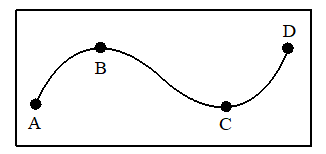The given figure shows a particle moving along a curve ABCD.Assertion (A) : The path followed by the particle is ABCDReason (R) : If we add the successive positions of the particle, we get the path of the particle

# The given figure shows a particle moving along a curve ABCD.Assertion (A) : The path followed by the particle is ABCDReason (R) : If we add the successive positions of the particle, we get the path of the particle

1. A

Both A and R are true and R is the correct explanation of the A

2. B

Both A and R are true but R is not the correct explanation of the A.

3. C

A is true,  R is false

4. D

A is false,  R is true

Register to Get Free Mock Test and Study Material

+91

Verify OTP Code (required)

### Solution:

From the figure

We can claim that Assertion is true that path of particles is along curve ABCD because we get.

path of a particle by tracing the successive positions of the particle which is in motion.

Here both Assertion (A) and Reason (R) are true and (R) is the correct explanation of (A).Register to Get Free Mock Test and Study Material

+91

Verify OTP Code (required)